# 为了电竞酒店的生意，我泡着枸杞整理了一份《00后黑话指南》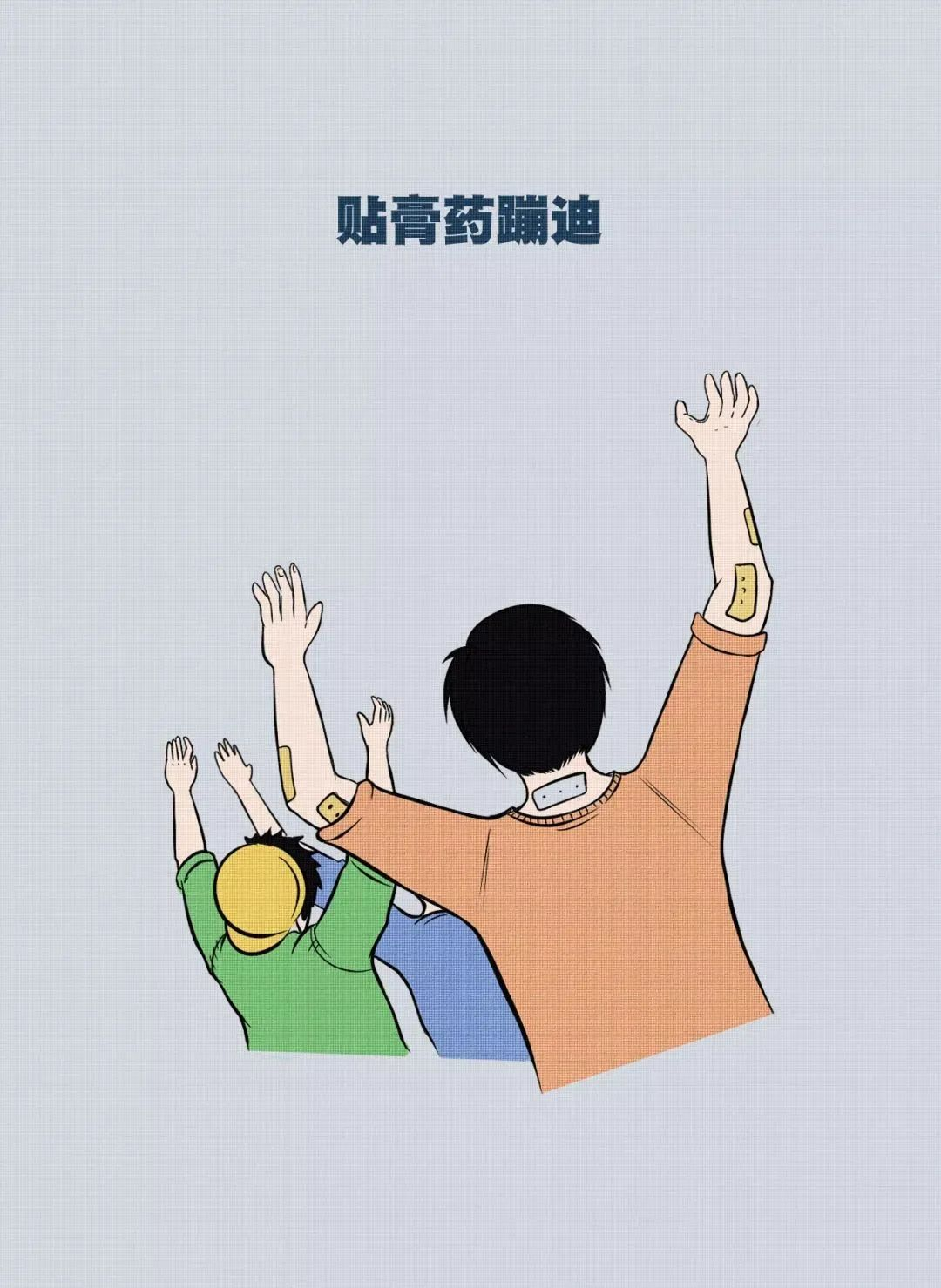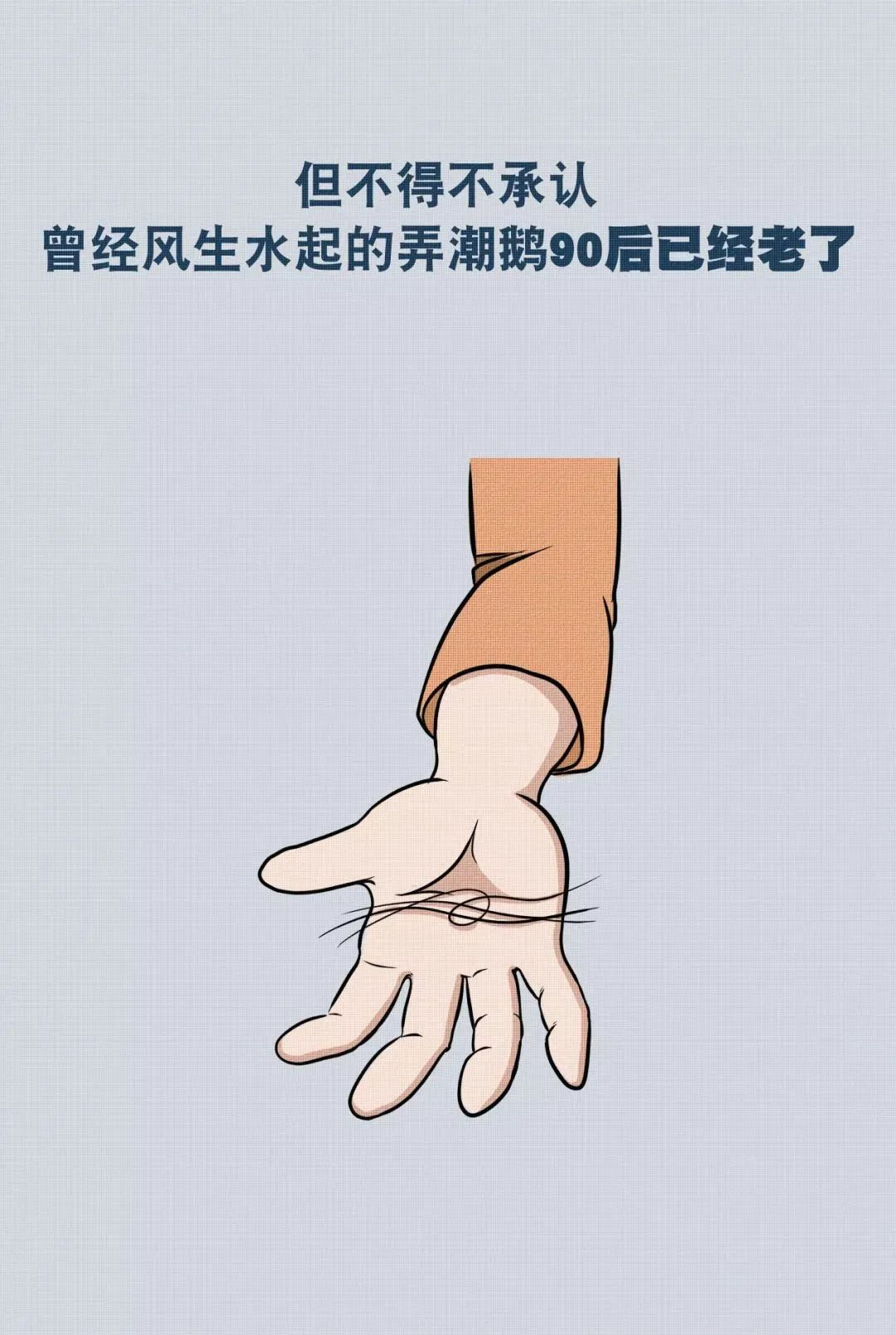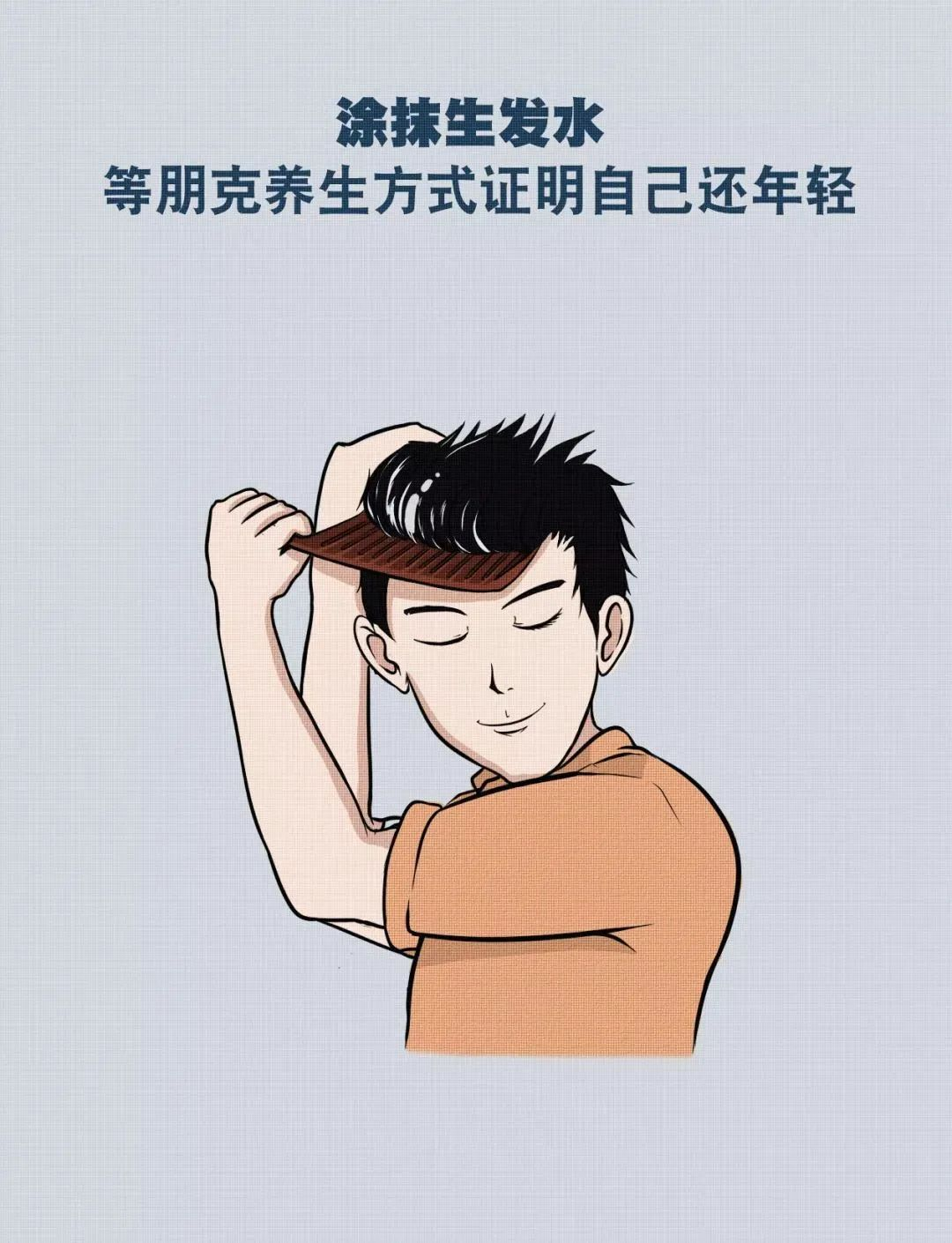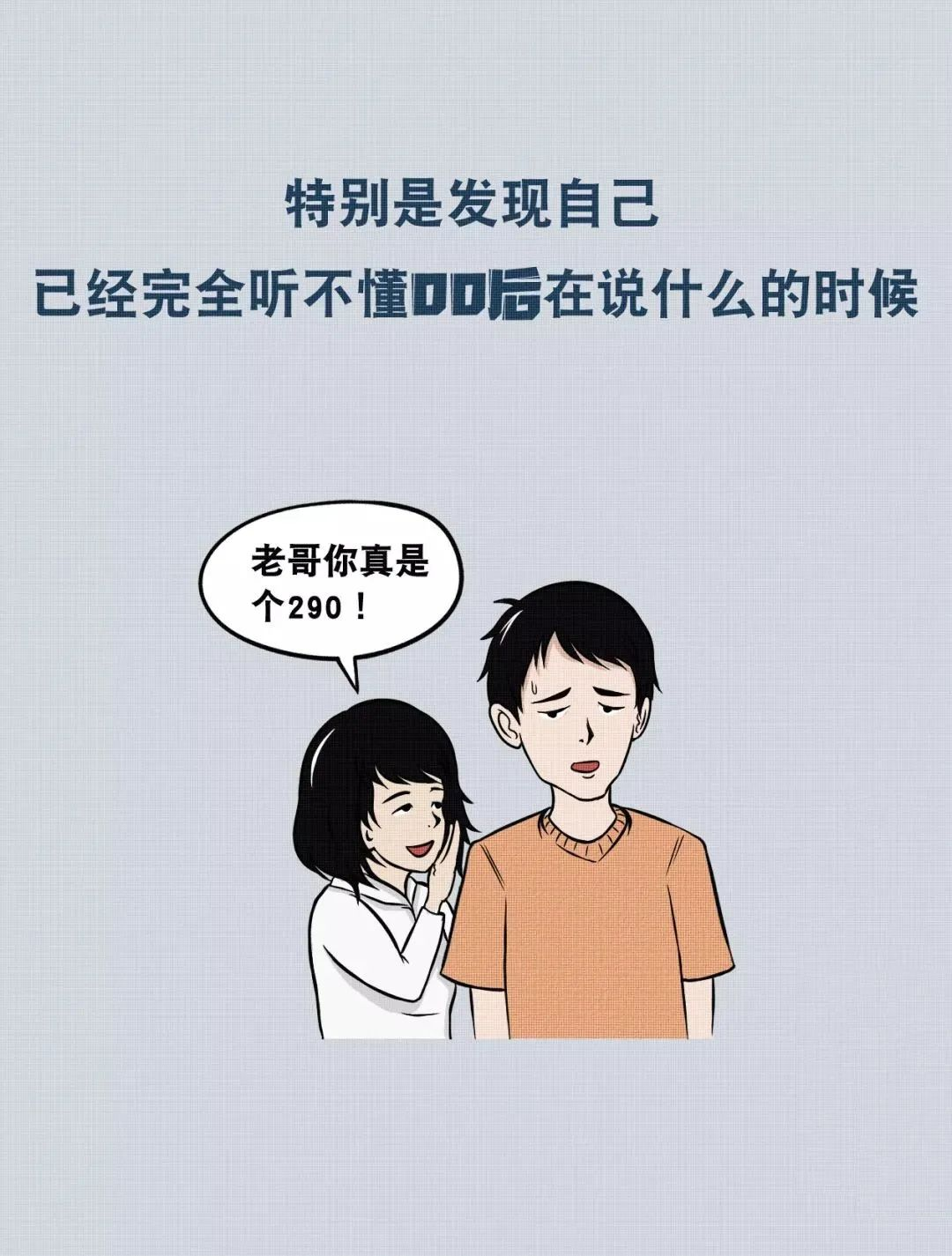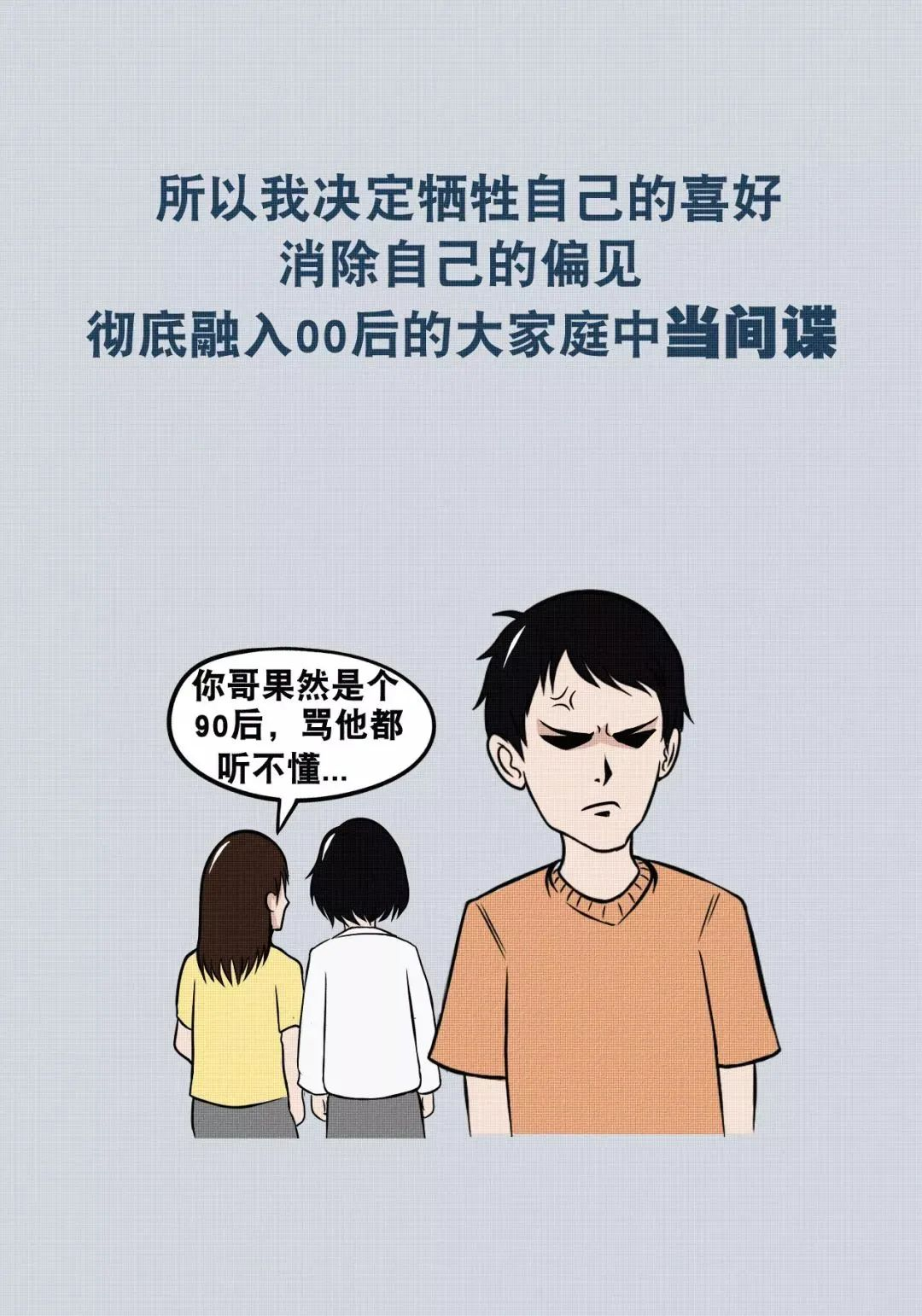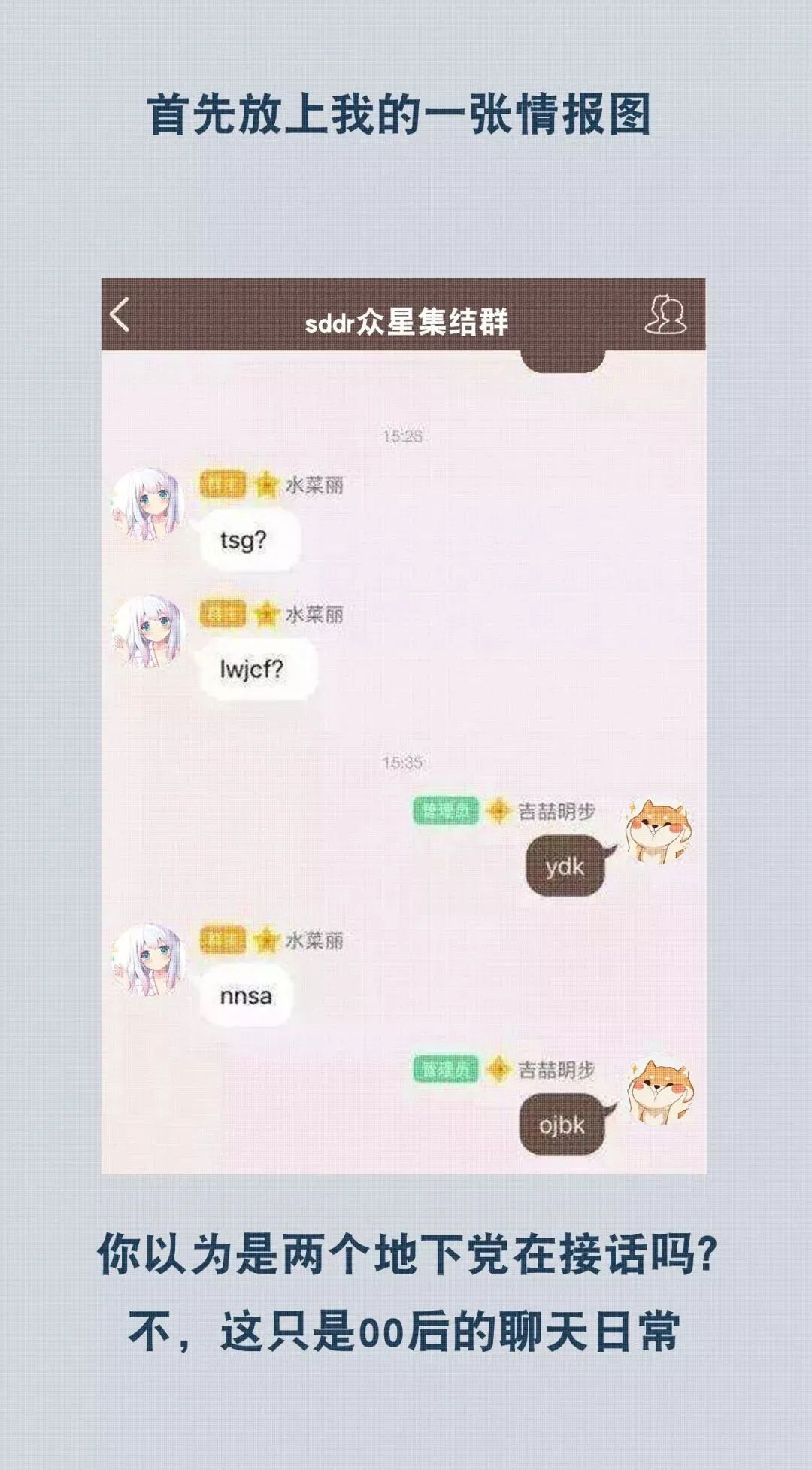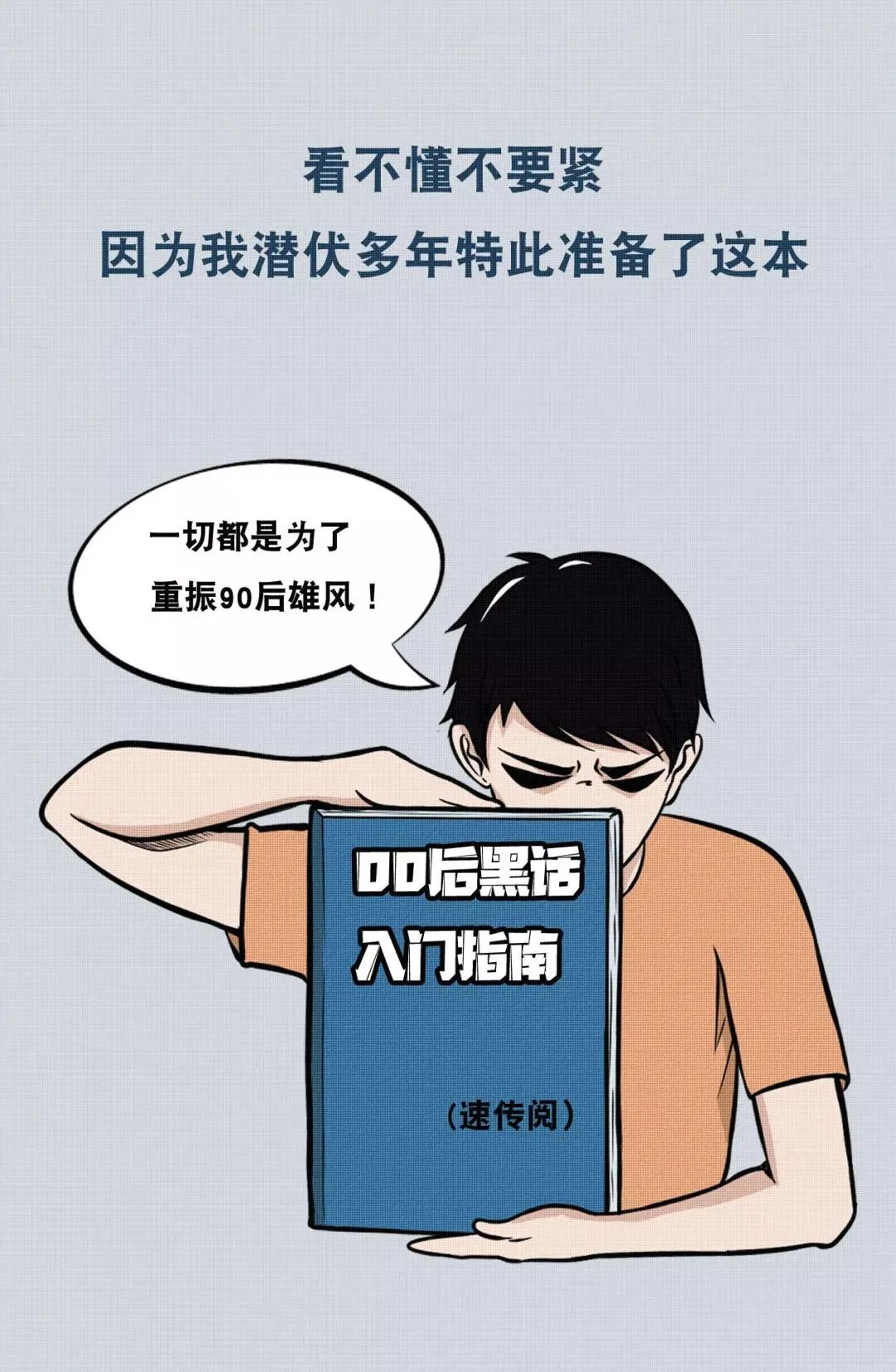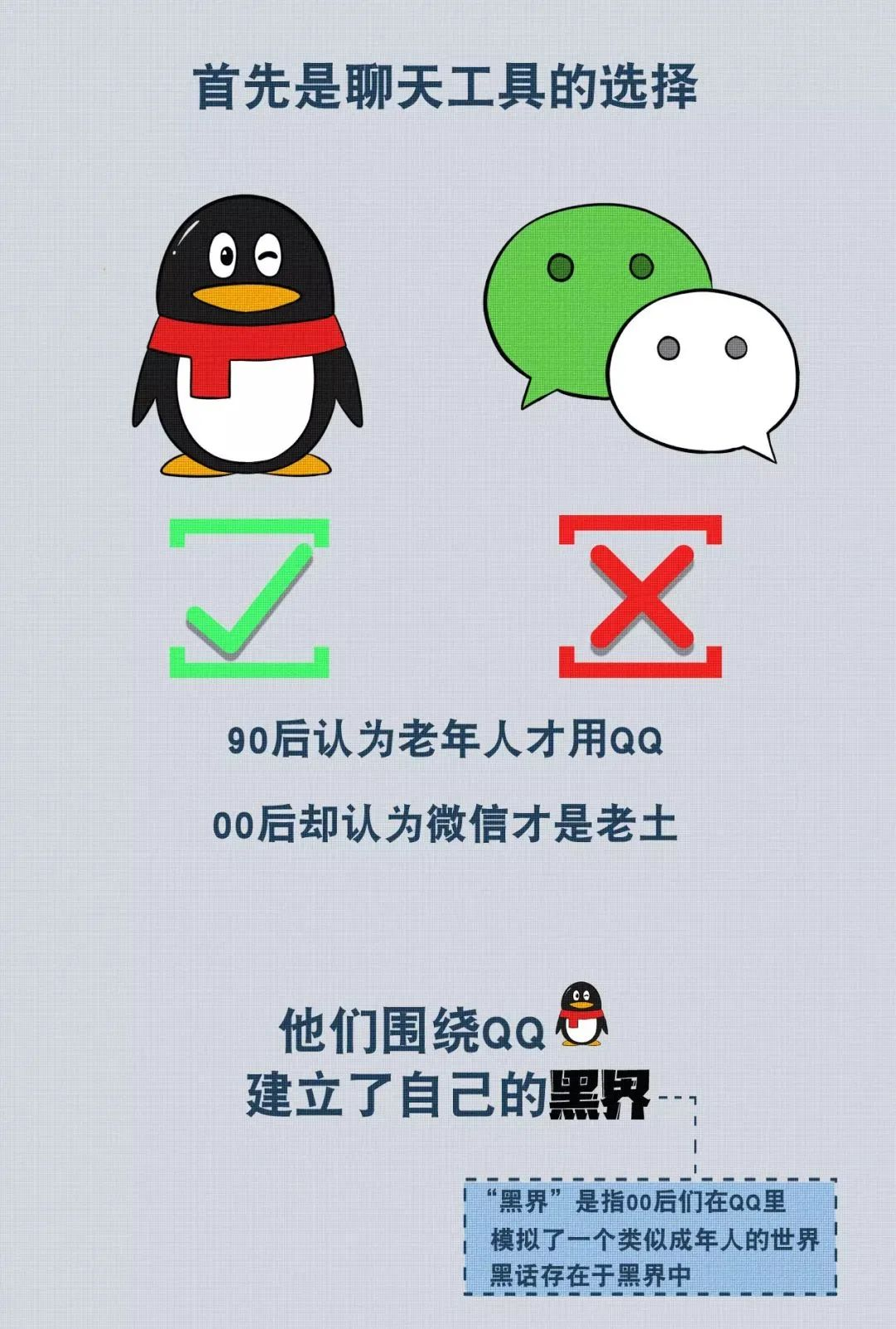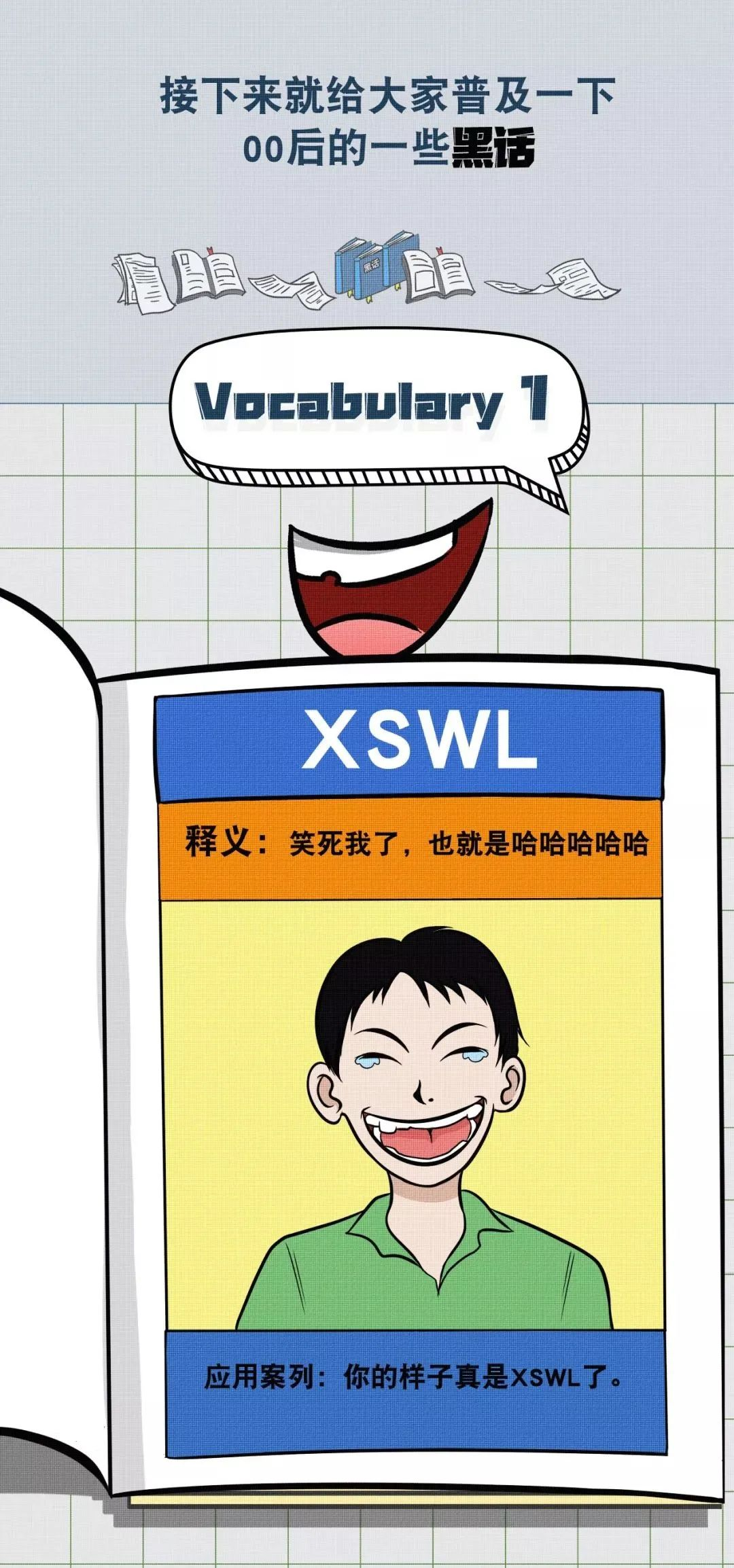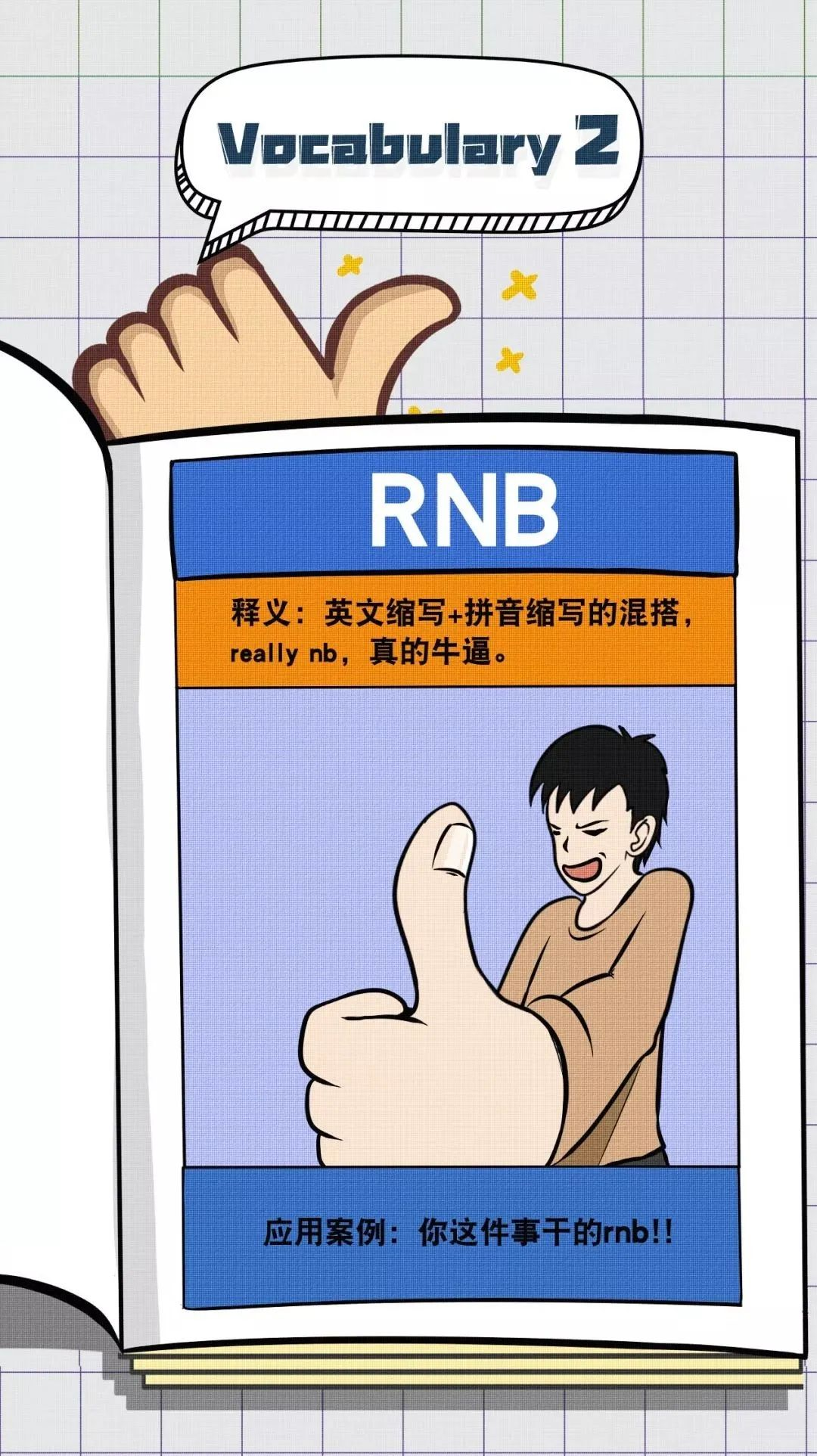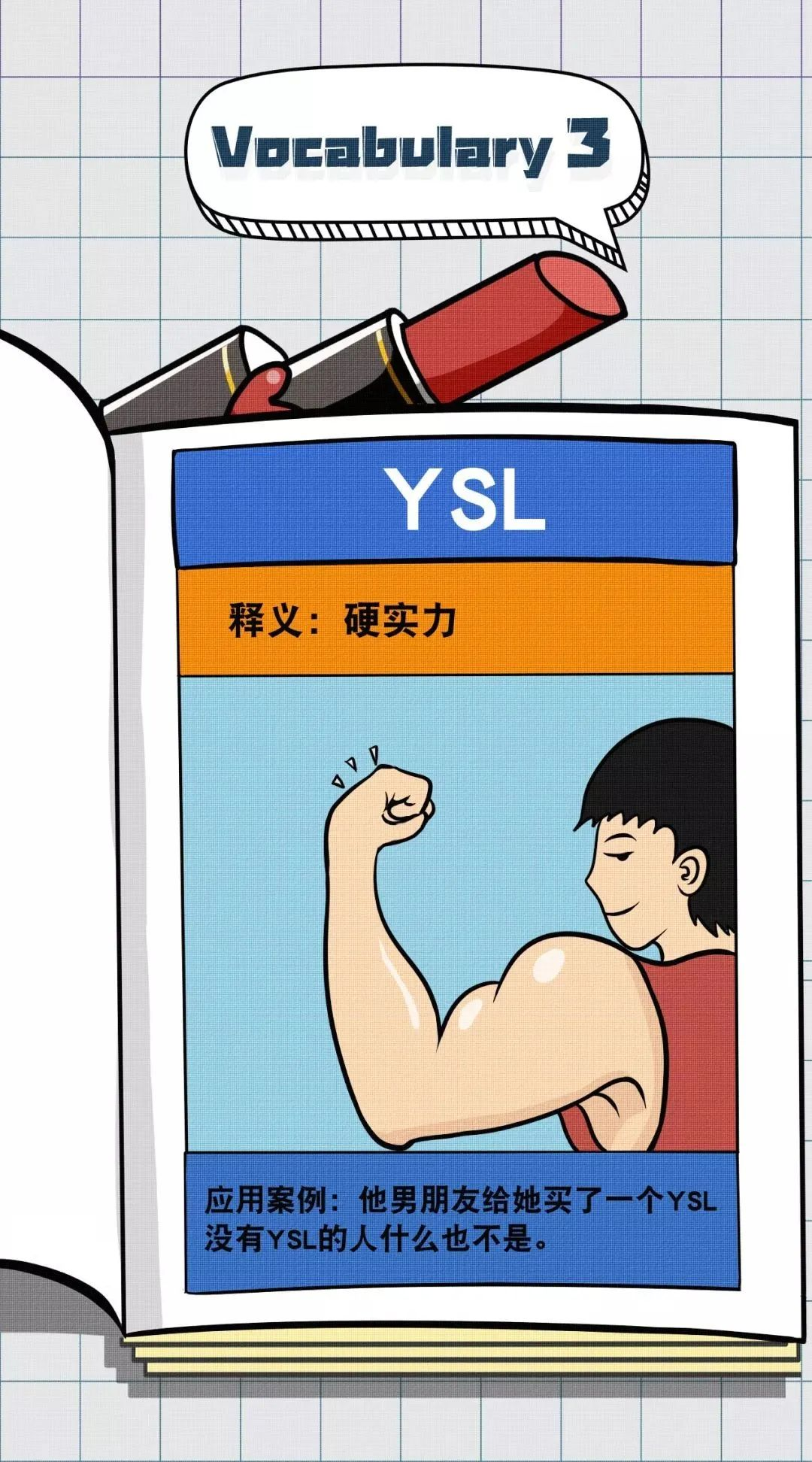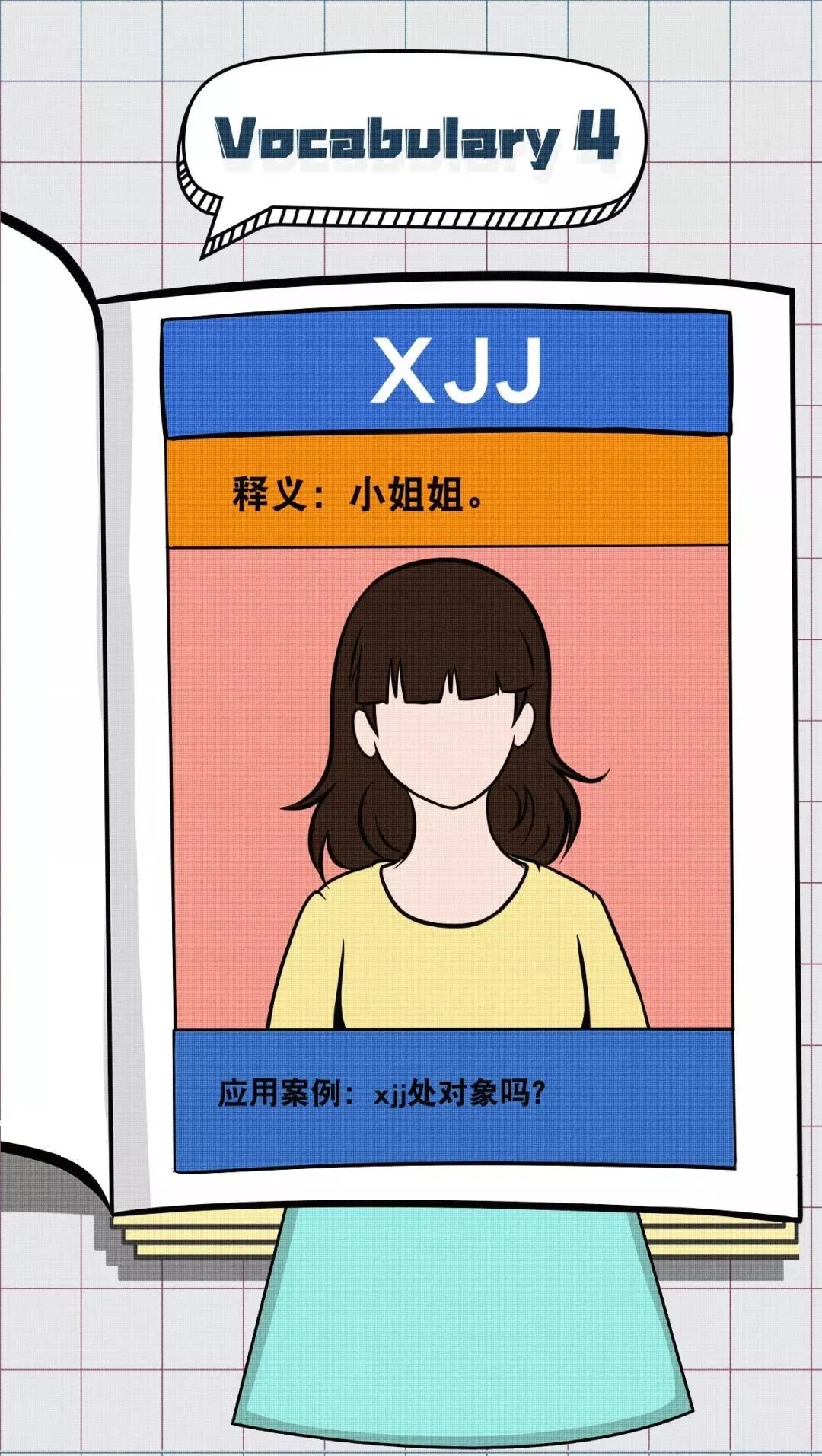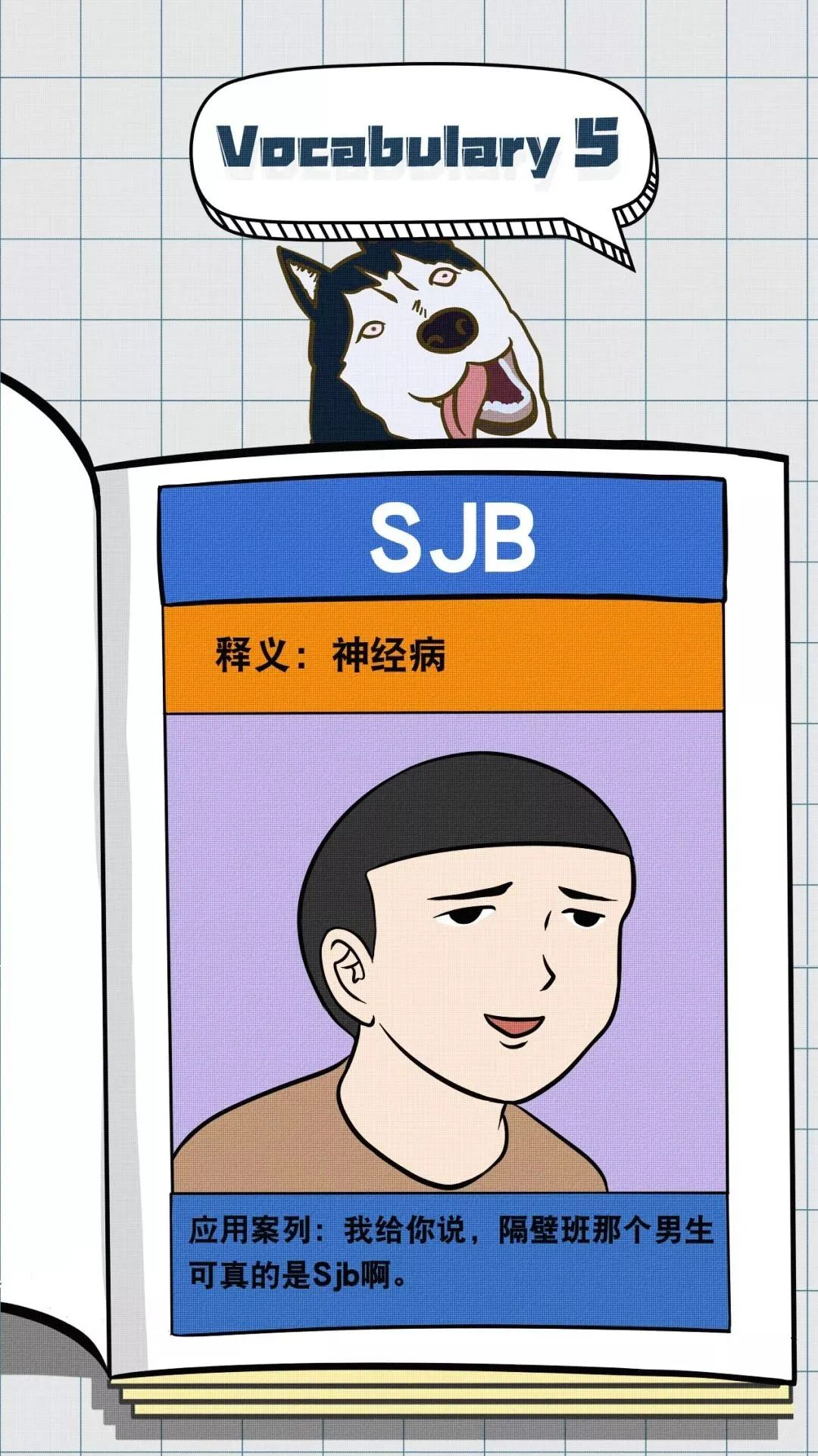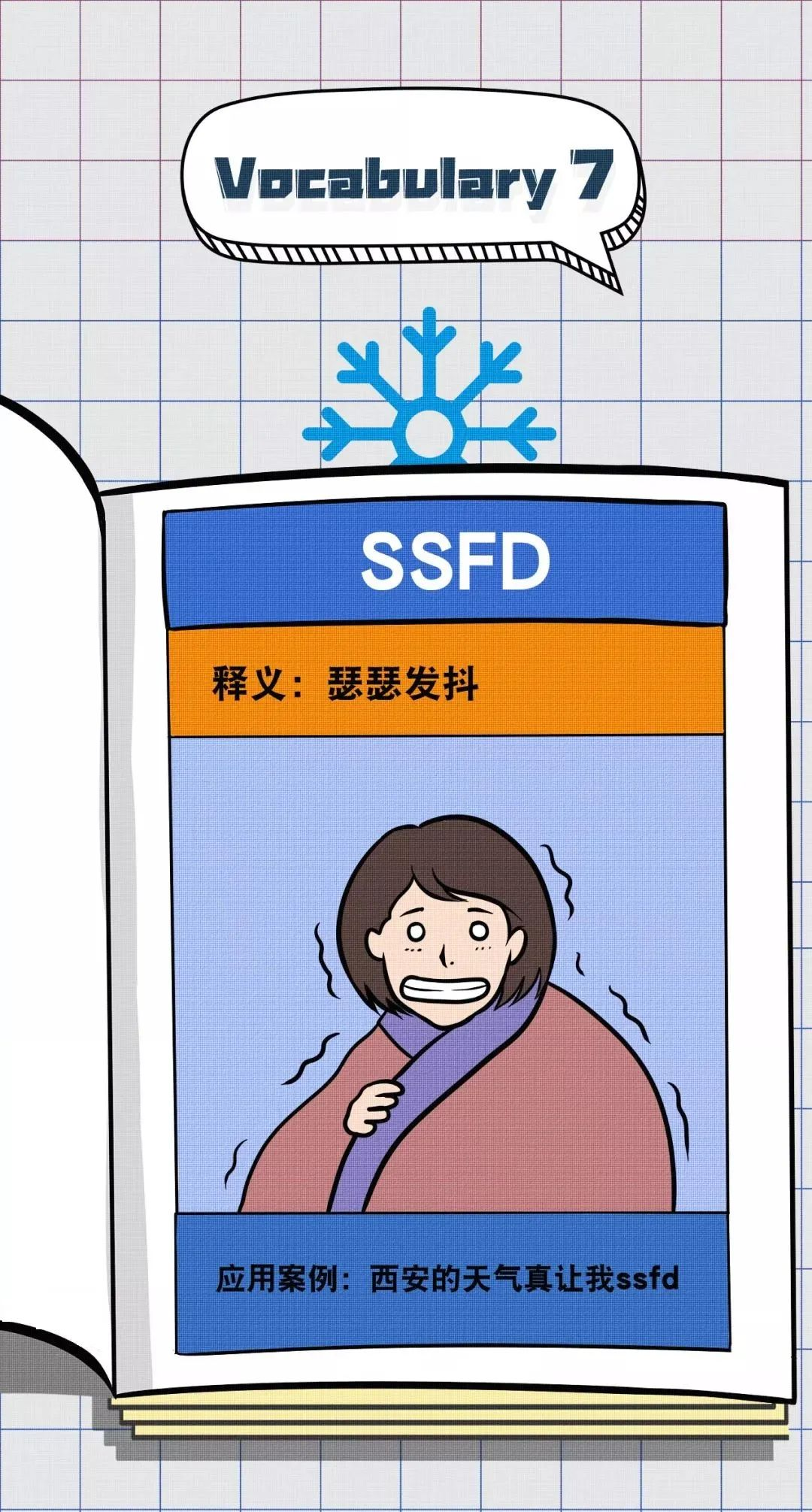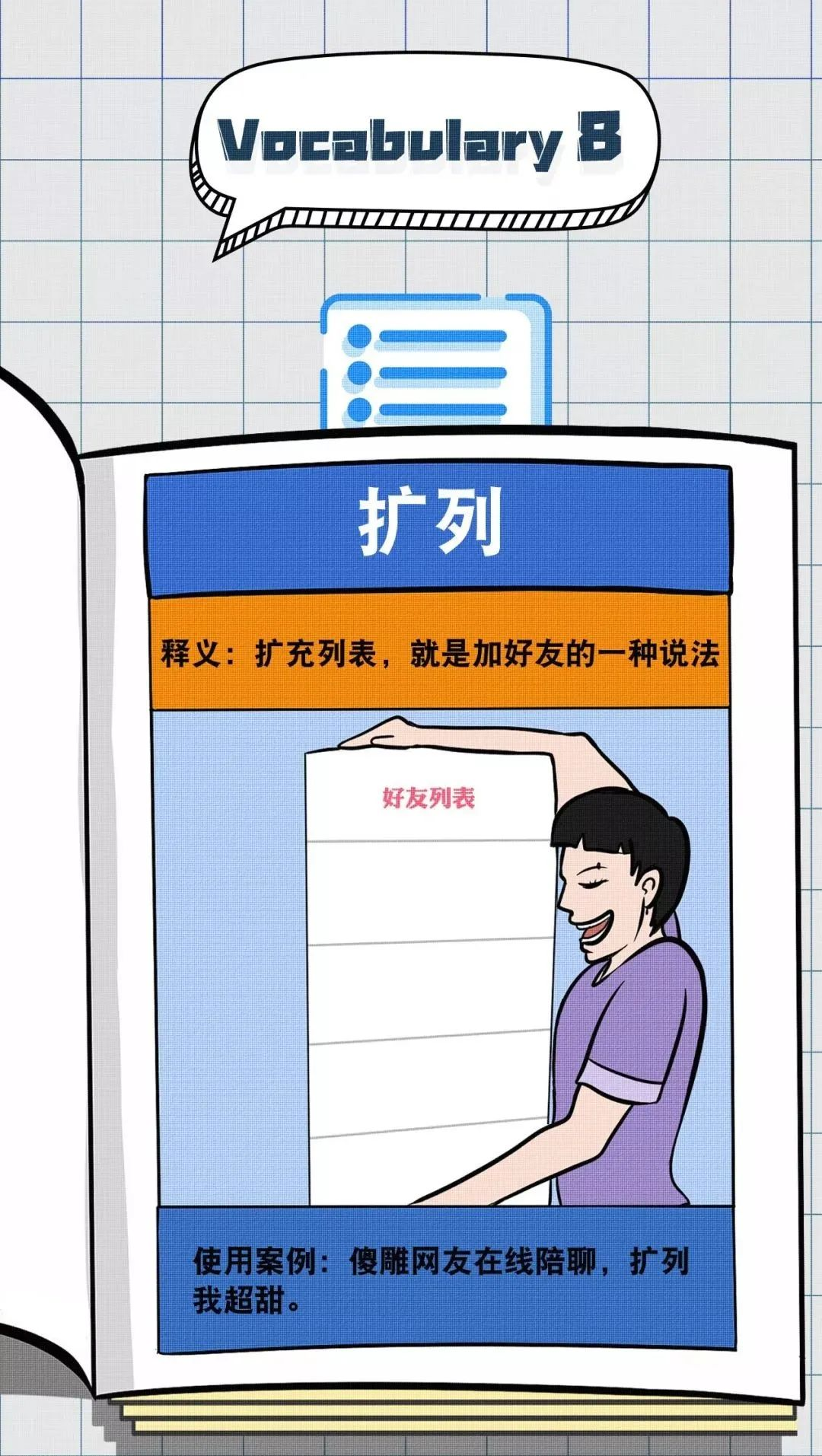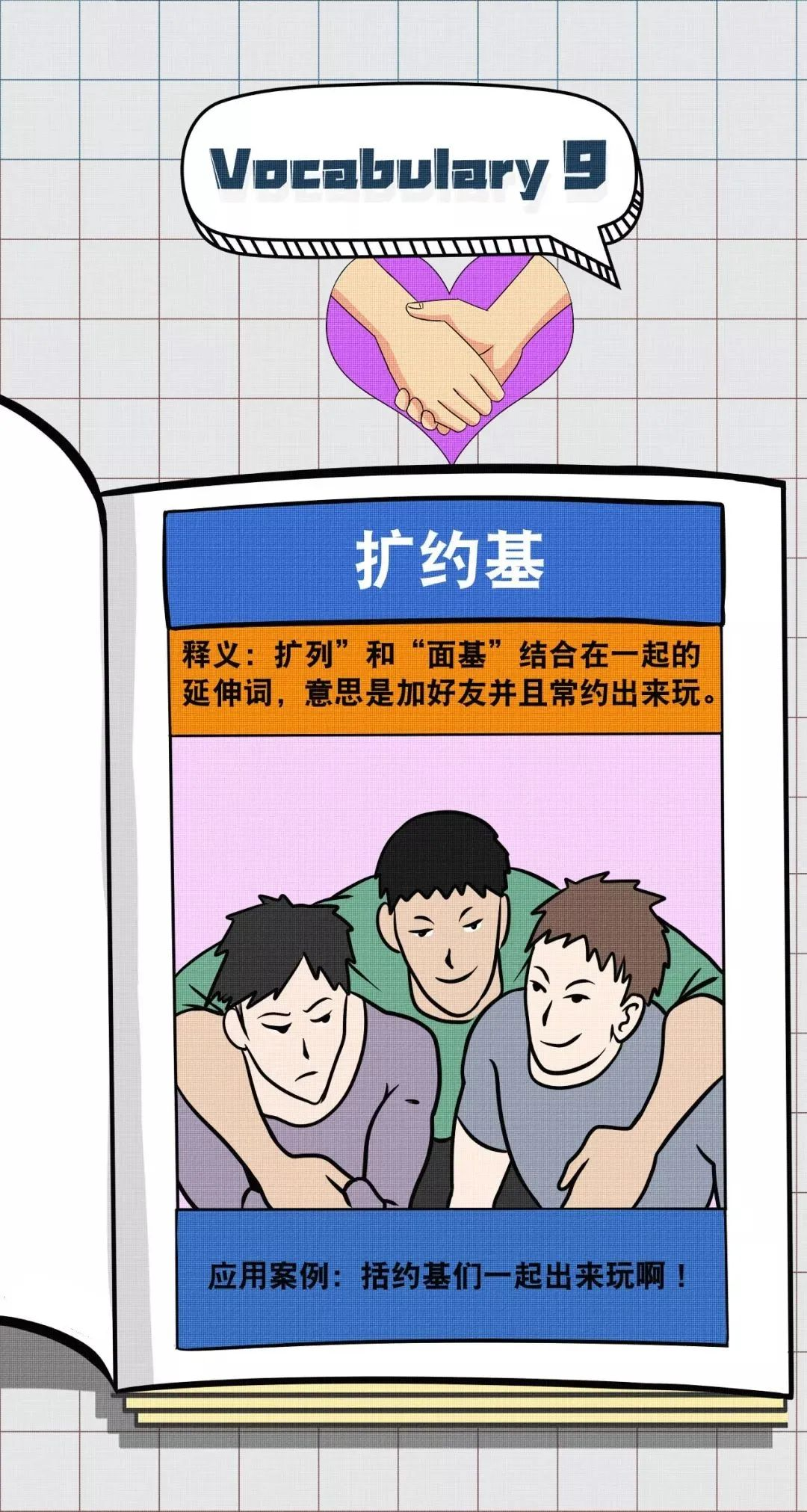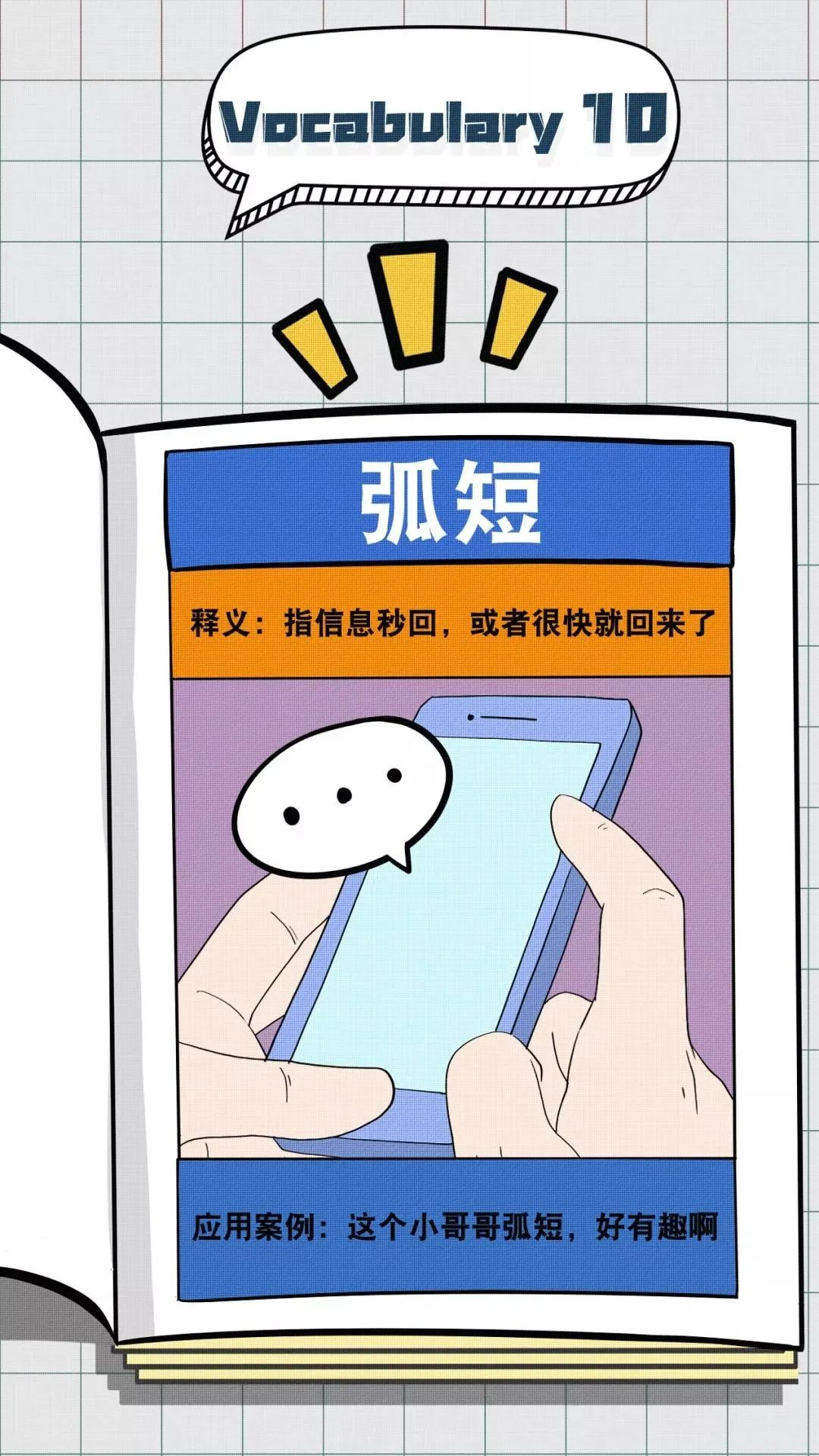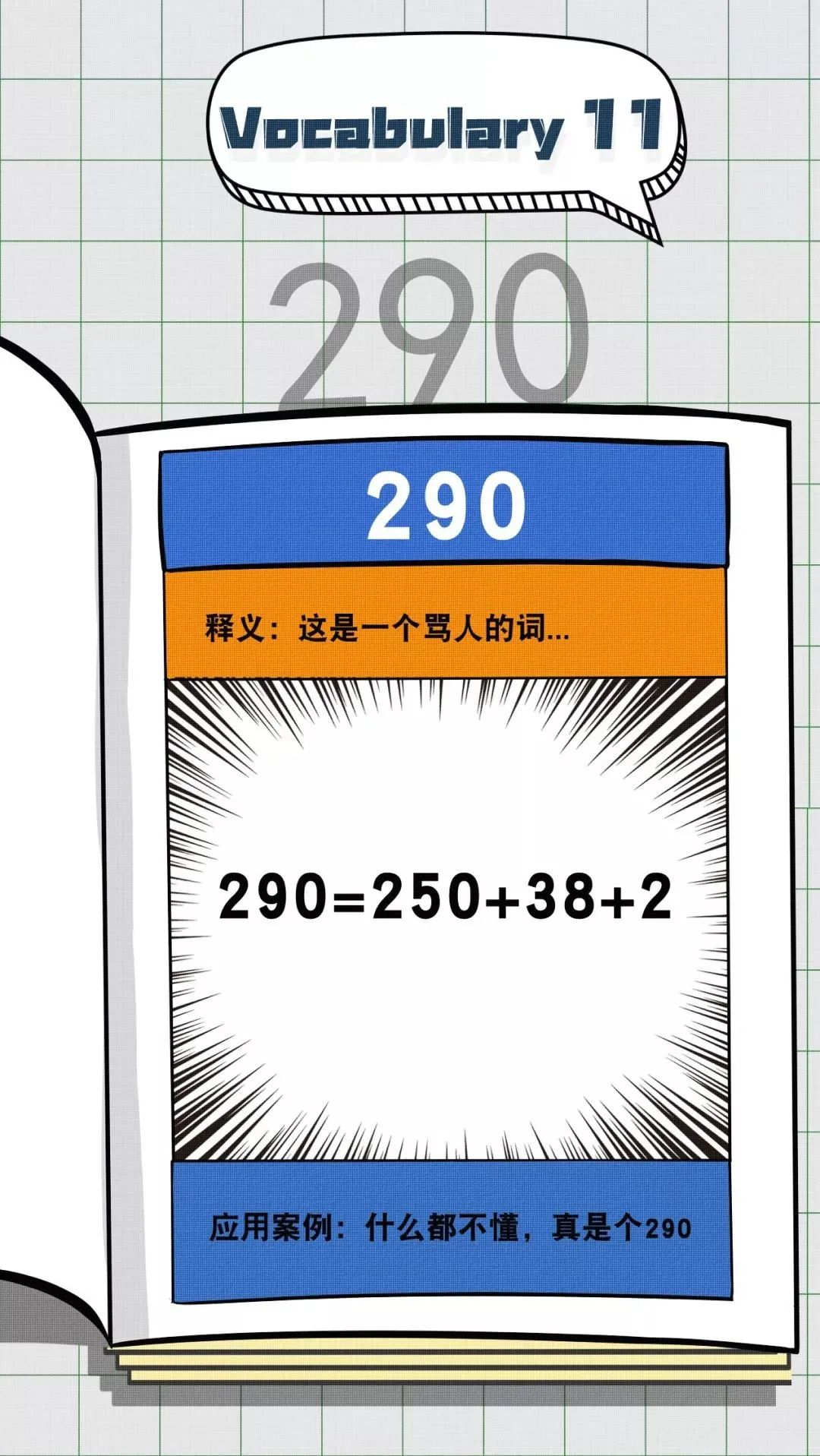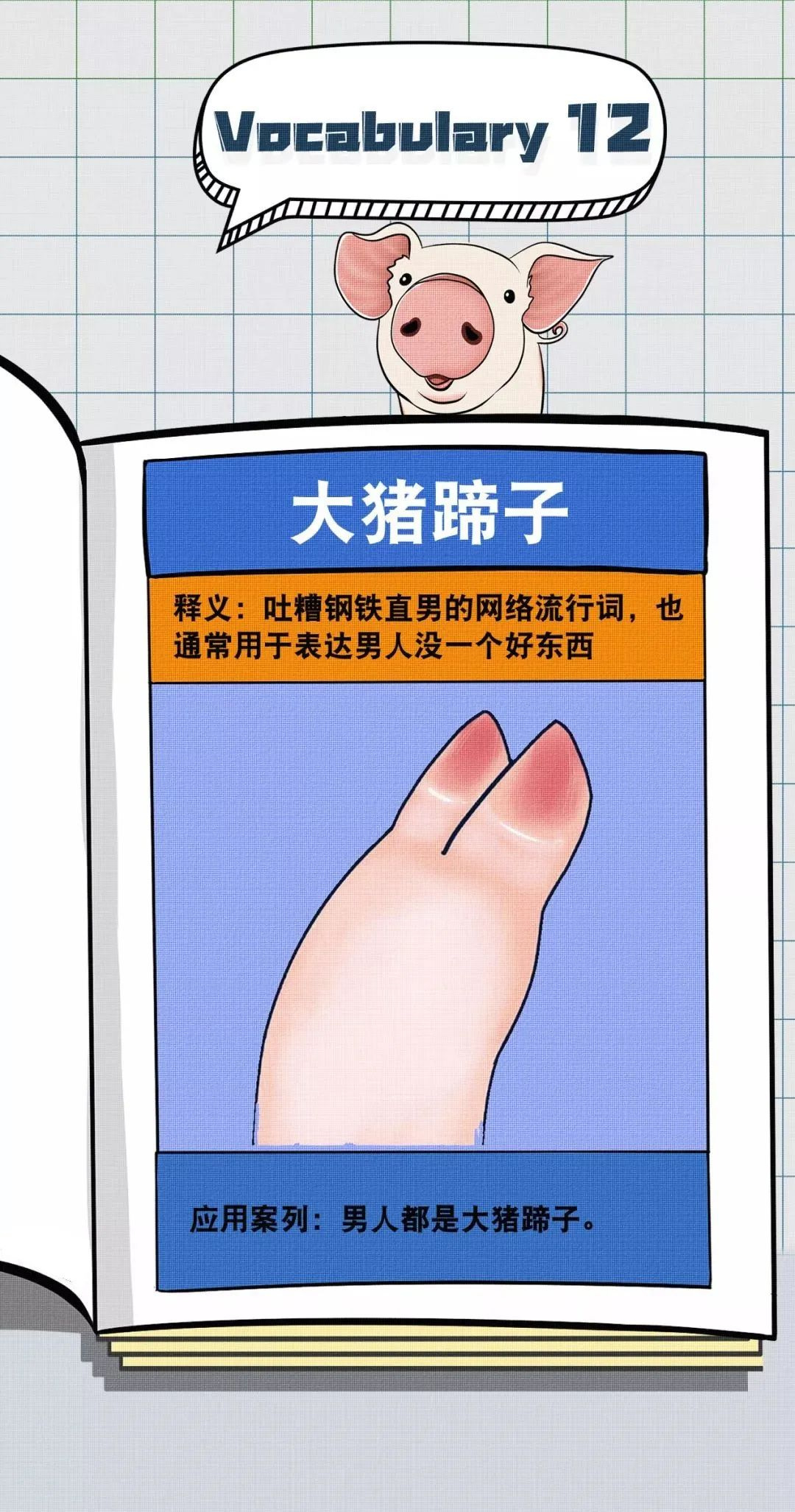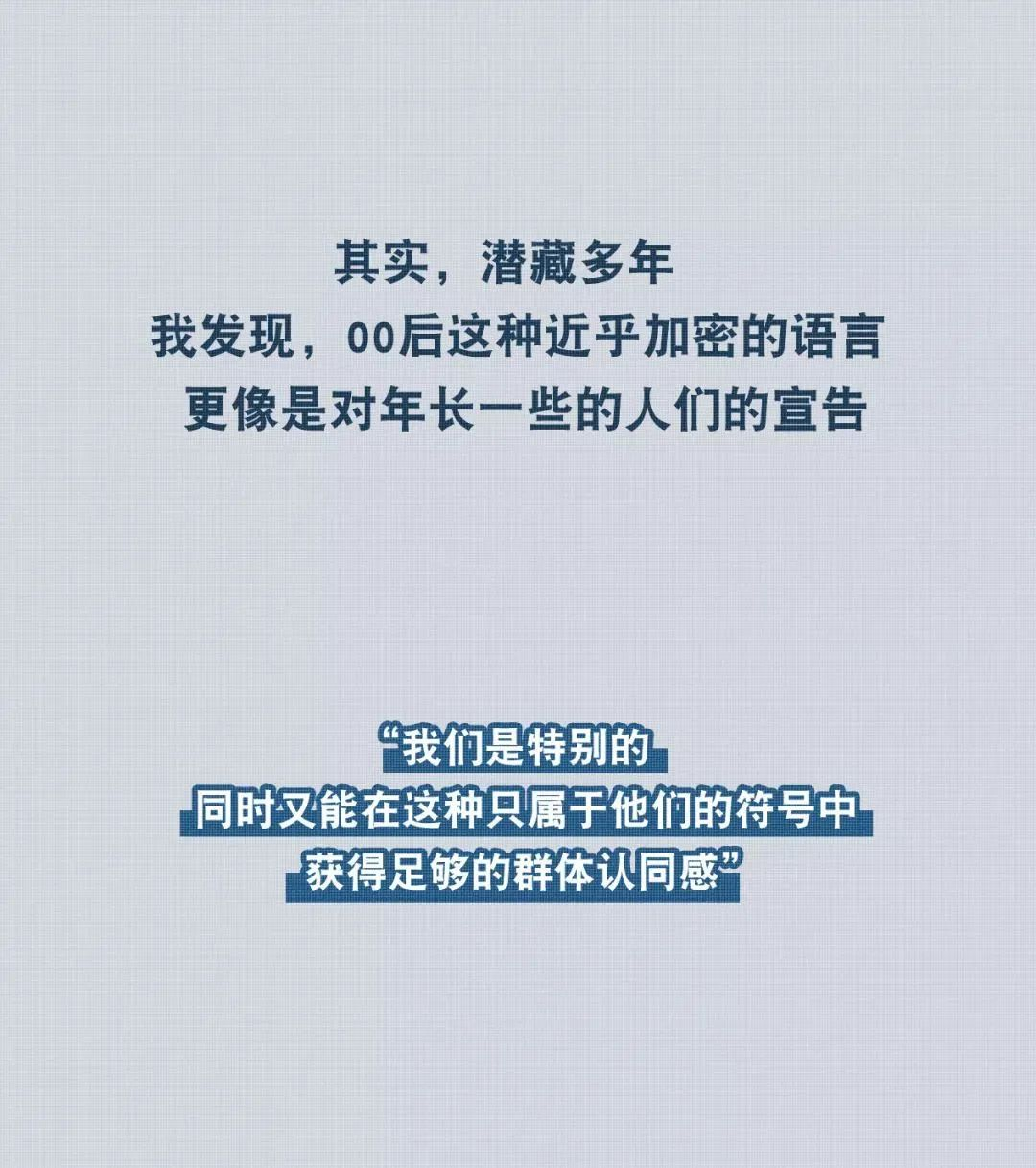mz=秒赞

nbcs=nobody cares没人关心的意思

yjgj=有句讲句，实话实说

dbq=对不起

bhys=不好意思

sk=生快（生日快乐的简称）

ylq=娱乐圈

myss=美颜盛世

ky=不会察言观色，没眼力劲

pph=扒皮黑，表面是粉丝，实质上是黑粉

pc=碰瓷

xxj=小学鸡

cpf=cp粉

bhs=不嗨森

rxl=real心累

nsdd=你说的对

djll=顶级流量

271=爱奇艺

txsp=腾讯视频

yk=优酷

lxs=练习生

zzr=制作人

hyh=后援会

awsl=（啊我死了）表达惊叹、佩服

nzbx=脑子不行

nzb=你走吧

cqy=处Q友

plmm=漂亮美妹

sqgg=帅气哥哥

ptsd=指看到某些字就自我高潮的人

hong了=表达疯了的意思

wwdmzzfyb=为我的莽撞自罚一杯

xyxf=血雨腥风